# NCERT Solutions for Class 6 Maths Chapter 4 Basic Geometrical Ideas (Ex 4.3) Exercise 4.3

## NCERT Solutions for Class 6 Maths Chapter 4 Basic Geometrical Ideas (Ex 4.3) Exercise 4.3

Free PDF download of NCERT Solutions for Class 6 Maths Chapter 4 Exercise 4.3 (Ex 4.3) and all chapter exercises at one place prepared by an expert teacher as per NCERT (CBSE) books guidelines. Class 6 Maths Chapter 4 Basic Geometrical Ideas Exercise 4.3 Questions with Solutions to help you to revise complete Syllabus and Score More marks. Register and get all exercise solutions in your emails.

Every NCERT Solution is provided to make the study simple and interesting on Vedantu. Subjects like Science, Maths, English, and Hindi will become easy to study if you have access to NCERT Solution for Class 6 Science, Maths solutions and solutions of other subjects.

Do you need help with your Homework? Are you preparing for Exams?
Study without Internet (Offline)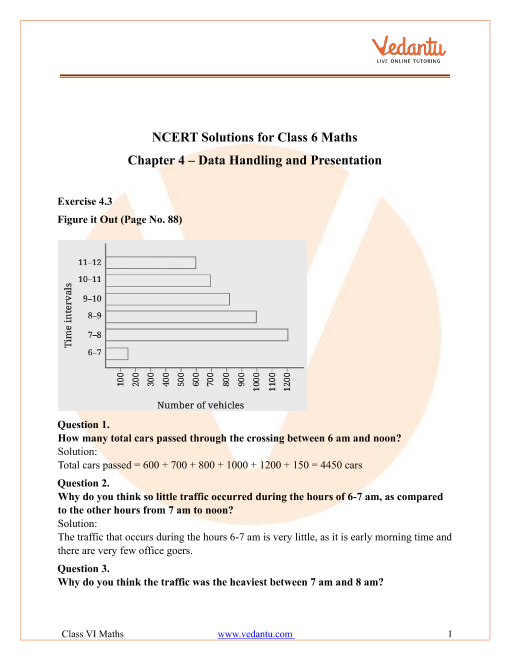Book your Free Demo session
Get a flavour of LIVE classes here at Vedantu## Access NCERT Solutions for Class 6 Maths Chapter 4 – Basic Geometric Ideas

Exercise 4.3

1. Name the angle in the given figure.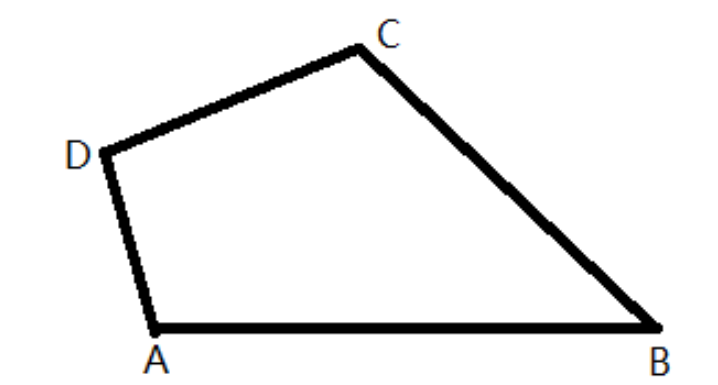Ans: An angle gets formed by two rays (or lines) and a common endpoint in the plane. The common point of the lines $CD$ and $DA$is $D$. So, the angle formed between $CD$ and $DA$is $\angle CDA$

The common point of the lines $DA$ and $AB$is $A$. So, the angle formed between $DA$ and $AB$is $\angle DAB$.

The common point of the lines $AB$ and $BC$is $B$. So, the angle formed between $AB$ and $BC$is $\angle ABC$.

The common point of the lines $BC$ and $CD$is $C$. So, the angle formed between $BC$ and $CD$is $\angle BCD$.

There are four angles in the given figure that are$\angle ABC,\angle CDA,\angle DAB,\angle DCB$.

2. In the given diagram, name the point(s).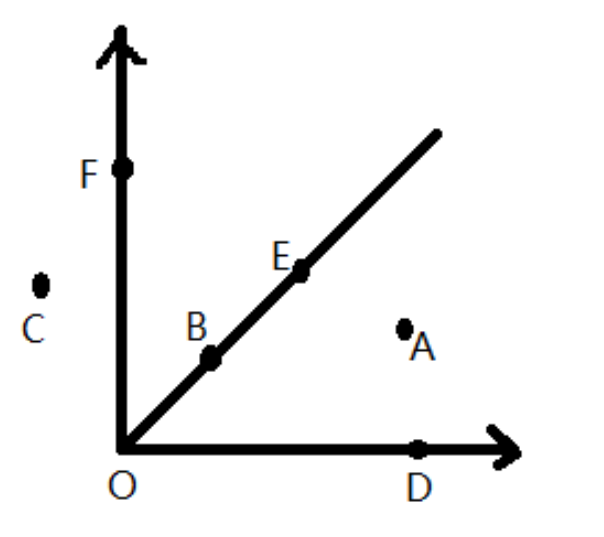(a) Name the point(s) in the interior of $\angle DOE$.

Ans: The points which lie within the arms of the angle when they are produced infinitely are called interior points of the angle. From the given graph, it can be seen that the point within the arms of the angle $\angle DOE$is $A$. So, the point $A$ is in the interior of $\angle DOE$.

(b) Name the point(s) in the exterior of $\angle EOF$.

Ans: The points which lie outside the arms of the angle produced infinitely are called exterior points of the angle. From the given graph, it can be seen that the points outside the arms of the angle $\angle EOF$are$C,A,D$. So, the points$C,A,D$ are the exterior of $\angle EOF$.

(c) Name the point(s) on$\angle EOF$.

Ans: From the given graph, it can be seen that the points on the arms of the angle $\angle EOF$are $E,O,B,F$. So, the points $E,O,B,F$ are on$\angle EOF$.

3. Draw rough diagrams of two angles such that they have the following things in common.

(a) Draw rough diagrams of two angles such that they have one point in common.

Ans: The rough diagram of two angles such that they have one point in common is shown below.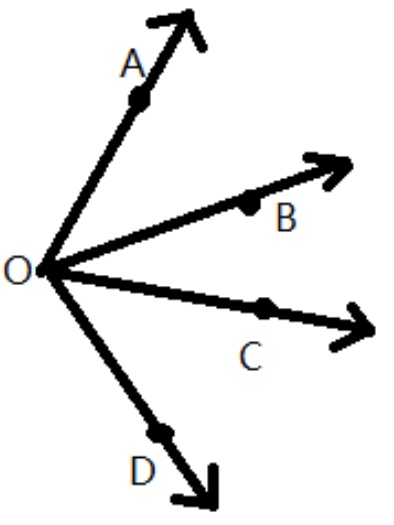Here, $\angle AOB$ and $\angle DOC$ have one common point, that is, $O$.

(b) Draw rough diagrams of two angles such that they have two points in common.

Ans: The rough diagram of two angles such that they have two points in common is shown below.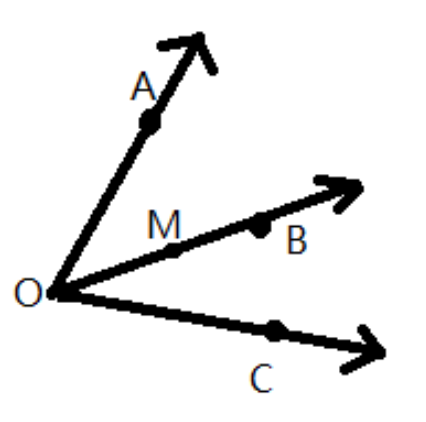Here, $\angle AOB$ and $\angle BOC$ have two common points that are, $O$ and $M$.

(c) Draw rough diagrams of two angles such that they have three points in common.

Ans: The rough diagram of two angles such that they have three points in common is shown below.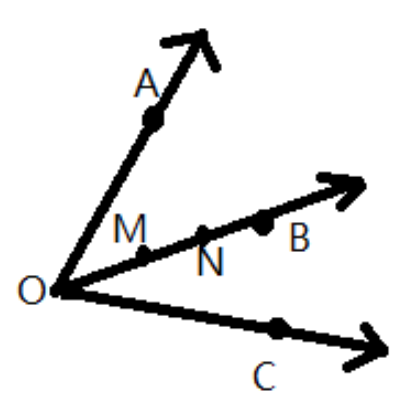Here, $\angle AOB$ and $\angle BOC$ have three common points that are, $O,N$ and $M$.

(d) Draw rough diagrams of two angles such that they have four points in common.

Ans: The rough diagram of two angles such that they have four points in common is shown below.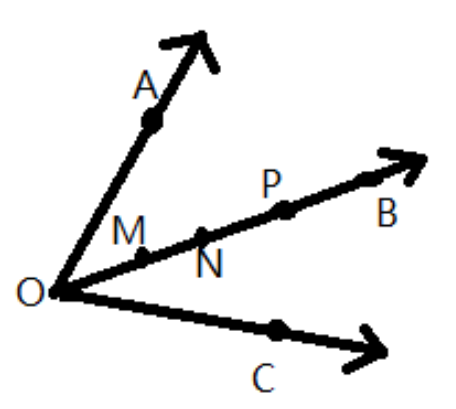Here, $\angle AOB$ and $\angle BOC$ have four common points that are, $O,N,P$ and $M$.

(e) Draw rough diagrams of two angles such that they have one ray in common.

Ans: The rough diagram of two angles such that they have one ray in common is shown below.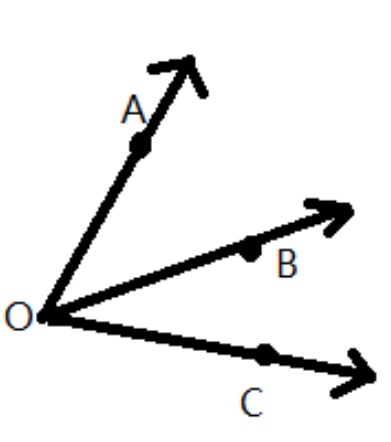Here, $\angle AOB$ and $\angle BOC$ have a ray in common that is $OB$.

## NCERT Solutions for Class 6 Maths Chapter 4 Basic Geometrical Ideas Exercise 4.3

Opting for the NCERT solutions for Ex 4.3 Class 6 Maths is considered as the best option for the CBSE students when it comes to exam preparation. This chapter consists of many exercises. Out of which we have provided the Exercise 4.3 Class 6 Maths NCERT solutions on this page in PDF format. You can download this solution as per your convenience or you can study it directly from our website/ app online.

Vedantu in-house subject matter experts have solved the problems/ questions from the exercise with the utmost care and by following all the guidelines by CBSE. Class 6 students who are thorough with all the concepts from the Maths textbook and quite well-versed with all the problems from the exercises given in it, then any student can easily score the highest possible marks in the final exam. With the help of this Class 6 Maths Chapter 4 Exercise 4.3 solutions, students can easily understand the pattern of questions that can be asked in the exam from this chapter and also learn the marks weightage of the chapter. So that they can prepare themselves accordingly for the final exam.

Besides these NCERT solutions for Class 6 Maths Chapter 4 Exercise 4.3, there are plenty of exercises in this chapter which contain innumerable questions as well. All these questions are solved/answered by our in-house subject experts as mentioned earlier. Hence all of these are bound to be of superior quality and anyone can refer to these during the time of exam preparation. In order to score the best possible marks in the class, it is really important to understand all the concepts of the textbooks and solve the problems from the exercises given next to it.

Do not delay any more. Download the NCERT solutions for Class 6 Maths Chapter 4 Exercise 4.3 from Vedantu website now for better exam preparation. If you have the Vedantu app in your phone, you can download the same through the app as well. The best part of these solutions is these can be accessed both online and offline as well.SHARETWEETSHARESUBSCRIBE